# LMS Collaborator knowledge base

Home/Content/Quizzes/Types of questions in quiz

## Types of questions in quiz

There are 7 types of questions that can be used in the quiz:

• single,
• multiple,
• ordering,
• classification,
• matching,
• select on image.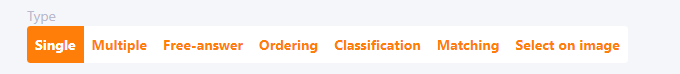#### «Single» question type

Single question. Contains an unlimited number of answer variants.
Only one answer variant is correct. While testing, the user can select only one answer variant as the correct answer.
If the user chooses the correct answer, he earns the number of points specified in the «Question Weight» parameter. Otherwise the user gets 0 points for the answer to the question.

Creating a «Single» type question: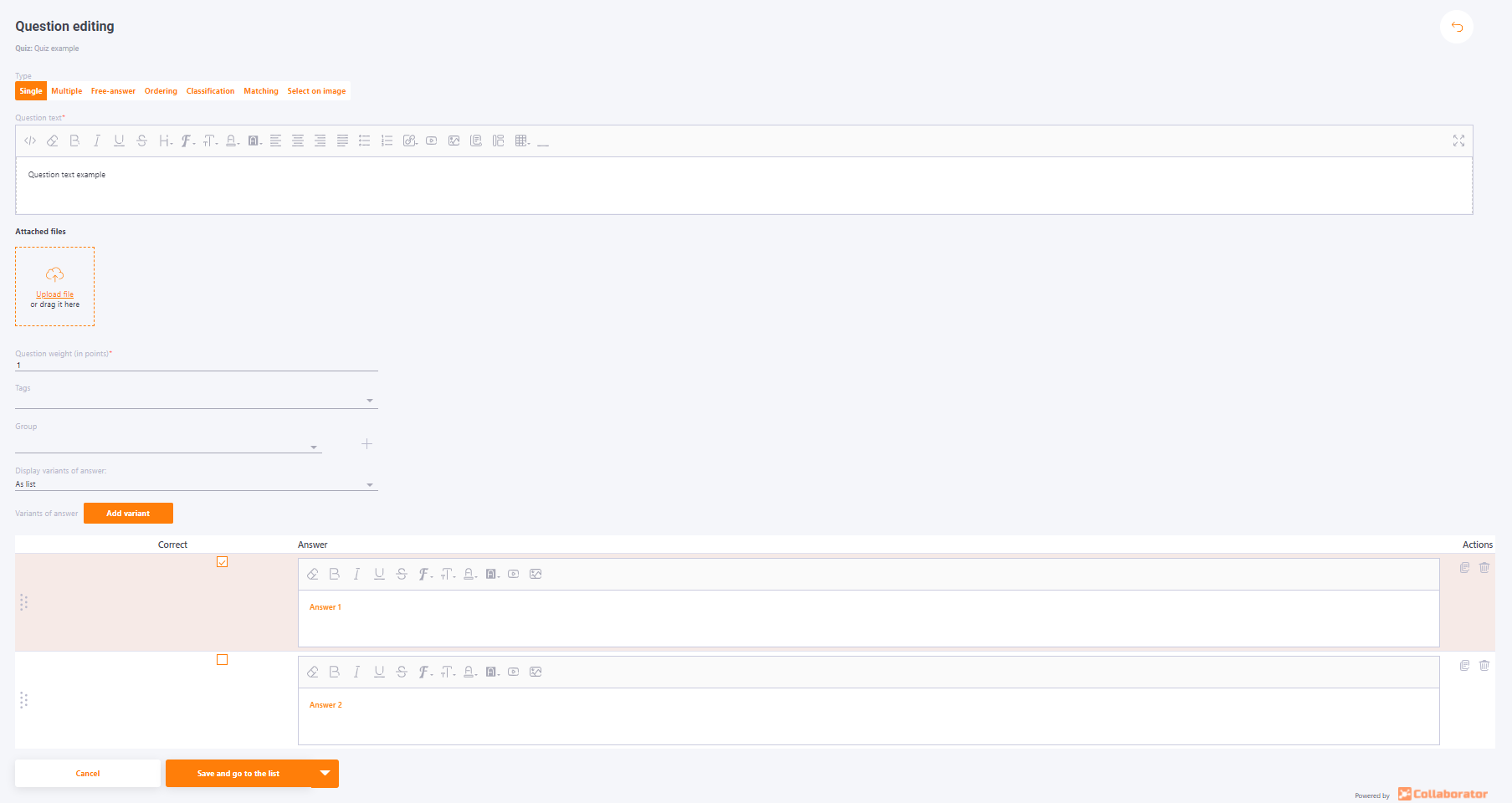#### "Multiple" question type

The question is of the closed type. Contains an unlimited number of answer variants.
One or more answer variants are correct. While testing, the user can select either one or more answer variants as correct answers.
Only when the user selects all correct answers and no wrong answers will they earn the number of points specified in the "Question Weight" parameter of the question.
For details on how the result of multiple choice questions is calculated, visit the following article Calculation of results for quizzes.

In the Answers number that could be selected field, a number is automatically set equal to the number of correct answers. But this number can be changed: it cannot be less than the number of correct answers and greater than the number of all answers to the question.

Creating a «Multiple» question type: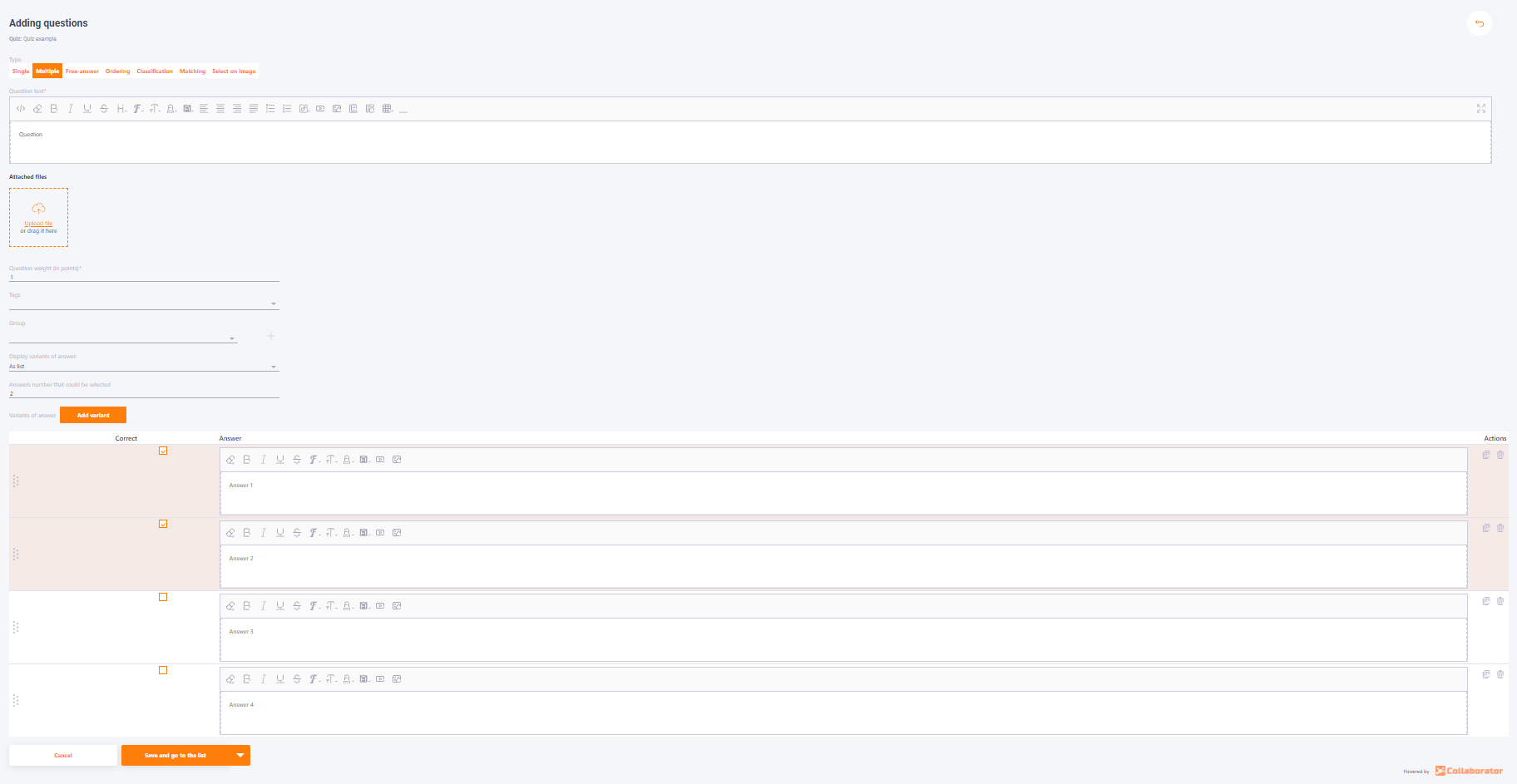#### Questions with a free answer

The "Free-answer" type of question assumes that the user writes their own answer. The user also has the ability to attach a file to the answer.
Allowed file formats that can be attached to the answer: jpg, png, jpeg, gif, xls, xlsx, doc, docx, zip, rar, 7z, pdf, mp4, flv, mp3.
The maximum size of the file that can be attached to the Free-answer question is 200 Mb.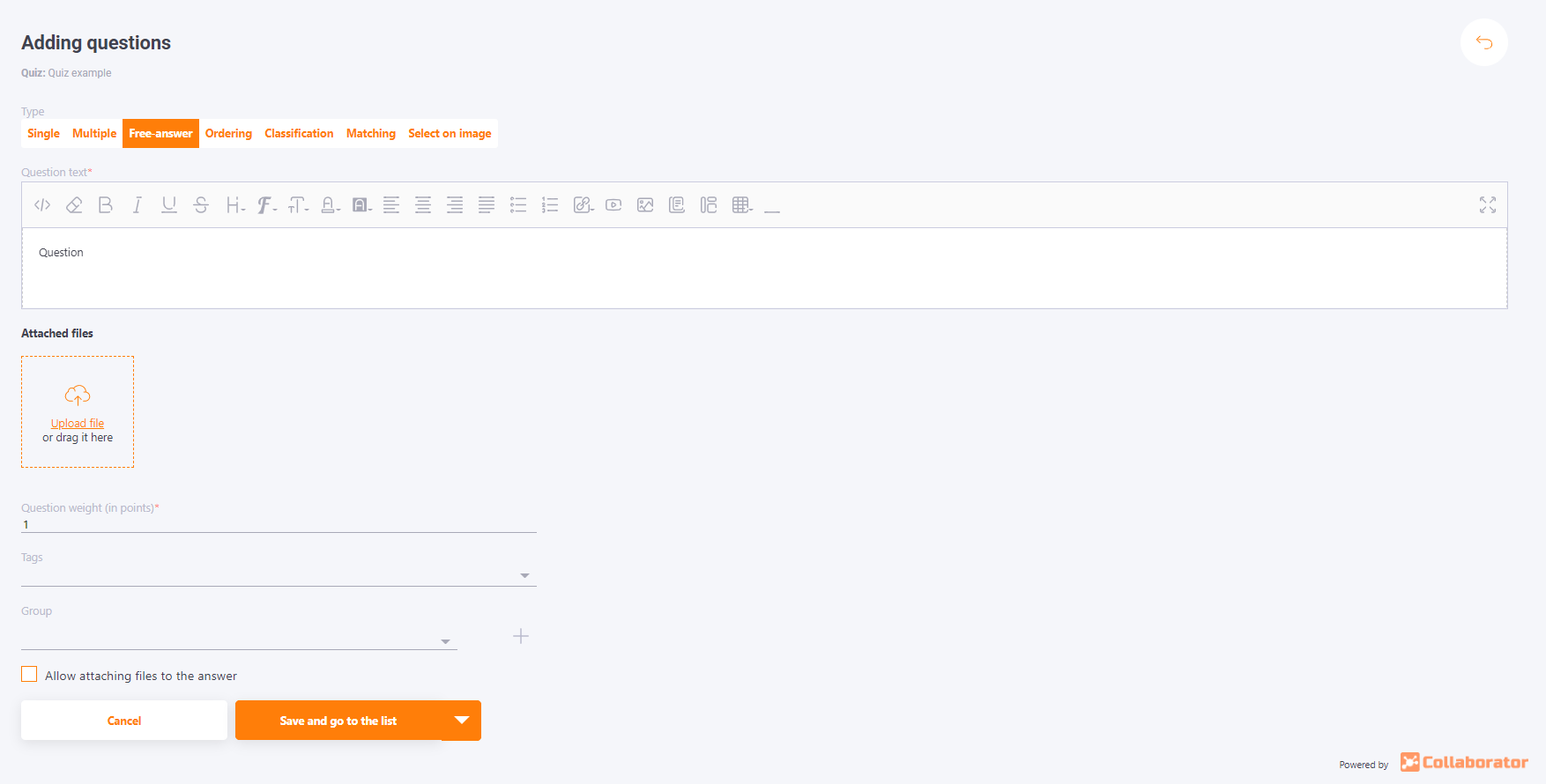After the answer is given, this type of question requires a check by the Tutor.

A list of the free-answer questions received will be provided in the Moderation - Check Answers section (find more on - Answers for checking).

If you set the question weight to 1 point, then when checking such a question, you can choose two options: "Correct" or " Wrong".
If you set the weight of the question more than 1 point (for example, 5) when testing will need to evaluate the answer a certain number of points from a specified range (for example, from 0 to 5).

Allowed file formats that can be attached to the question: jpg, png, jpeg, gif, svg.

On the question creation page there is the Add correct answer hint for moderator checkbox.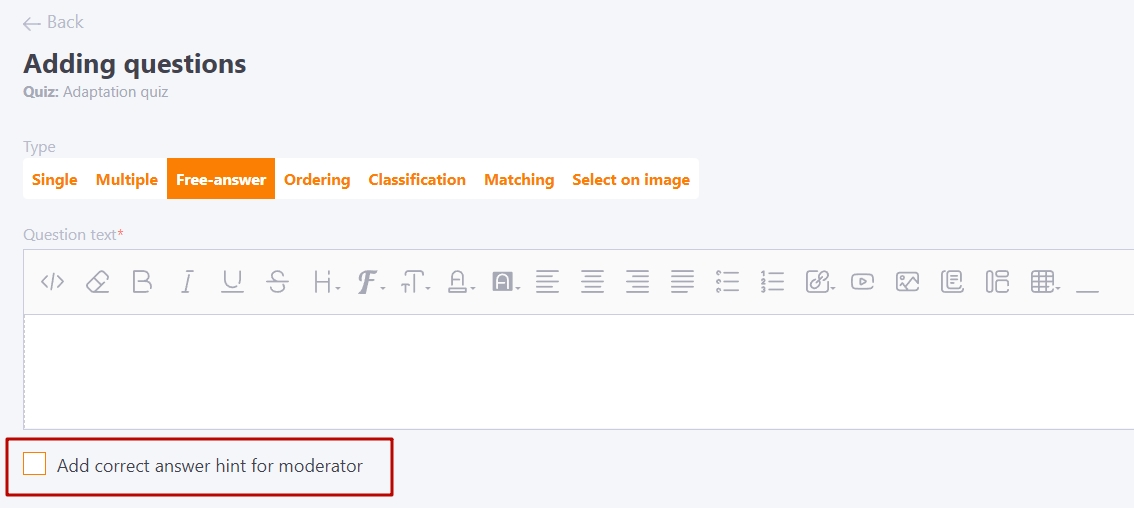If the checkbox is enabled, a field for entering the correct answer appears below it: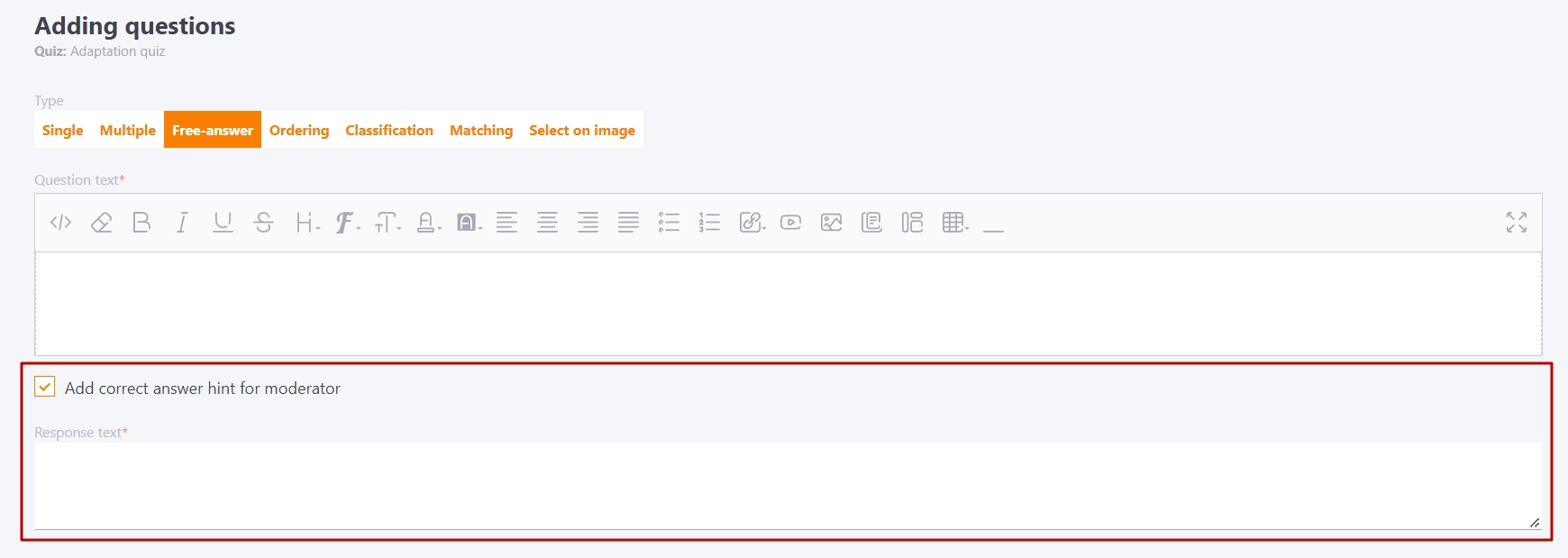#### Ordering questions

The "Ordering" type question involves arranging the answer variants in the correct order.

Creating the "Ordering" type question: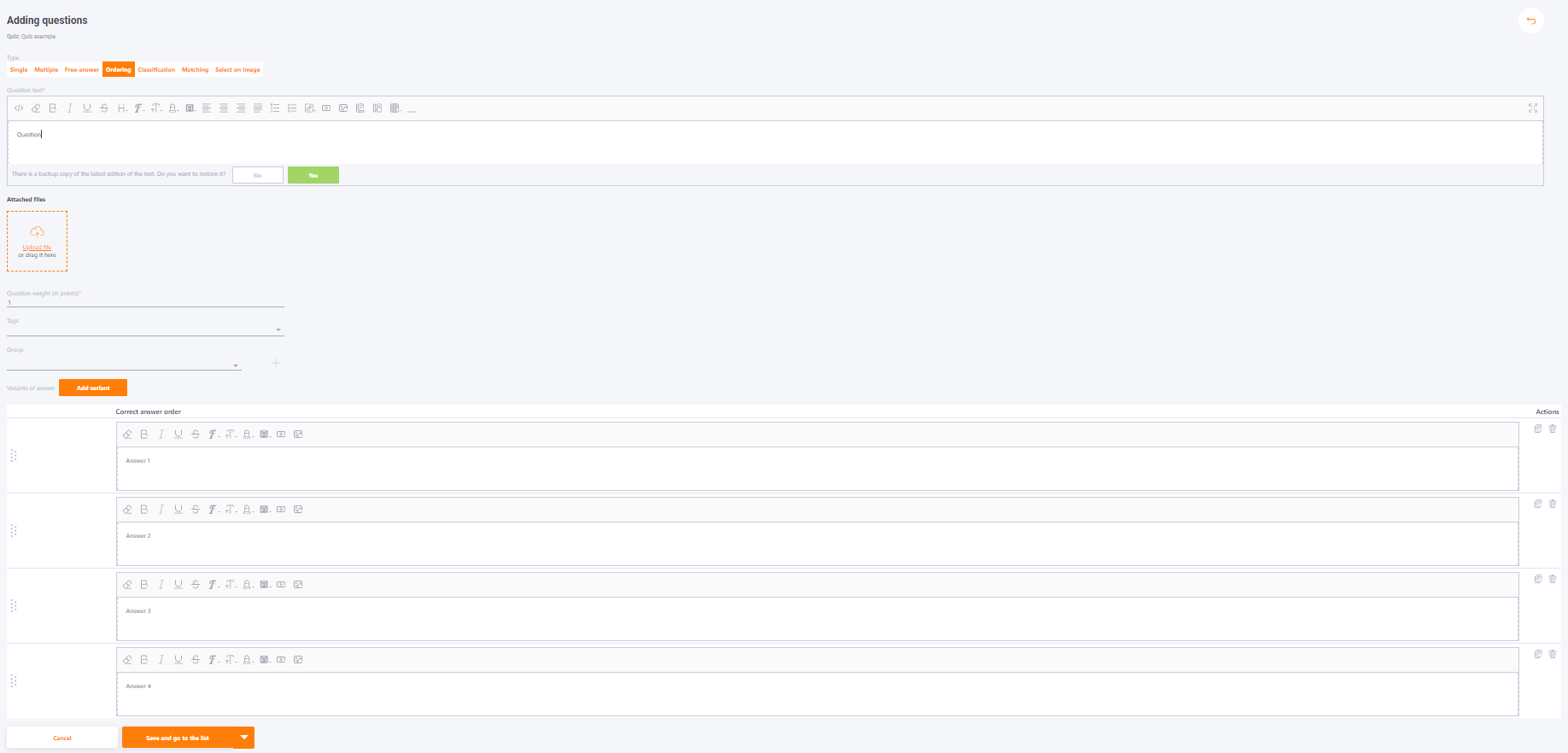While passing, the User will have to arrange the answer variants in the order in which they are arranged when creating the question.

Both the question and the answer options can be uploaded with pictures.

#### Classification questions

In this type of question, the user needs to assign answer variants to the suggested categories. Drag and drop the answer variants into the selected categories, similar to the ordering question.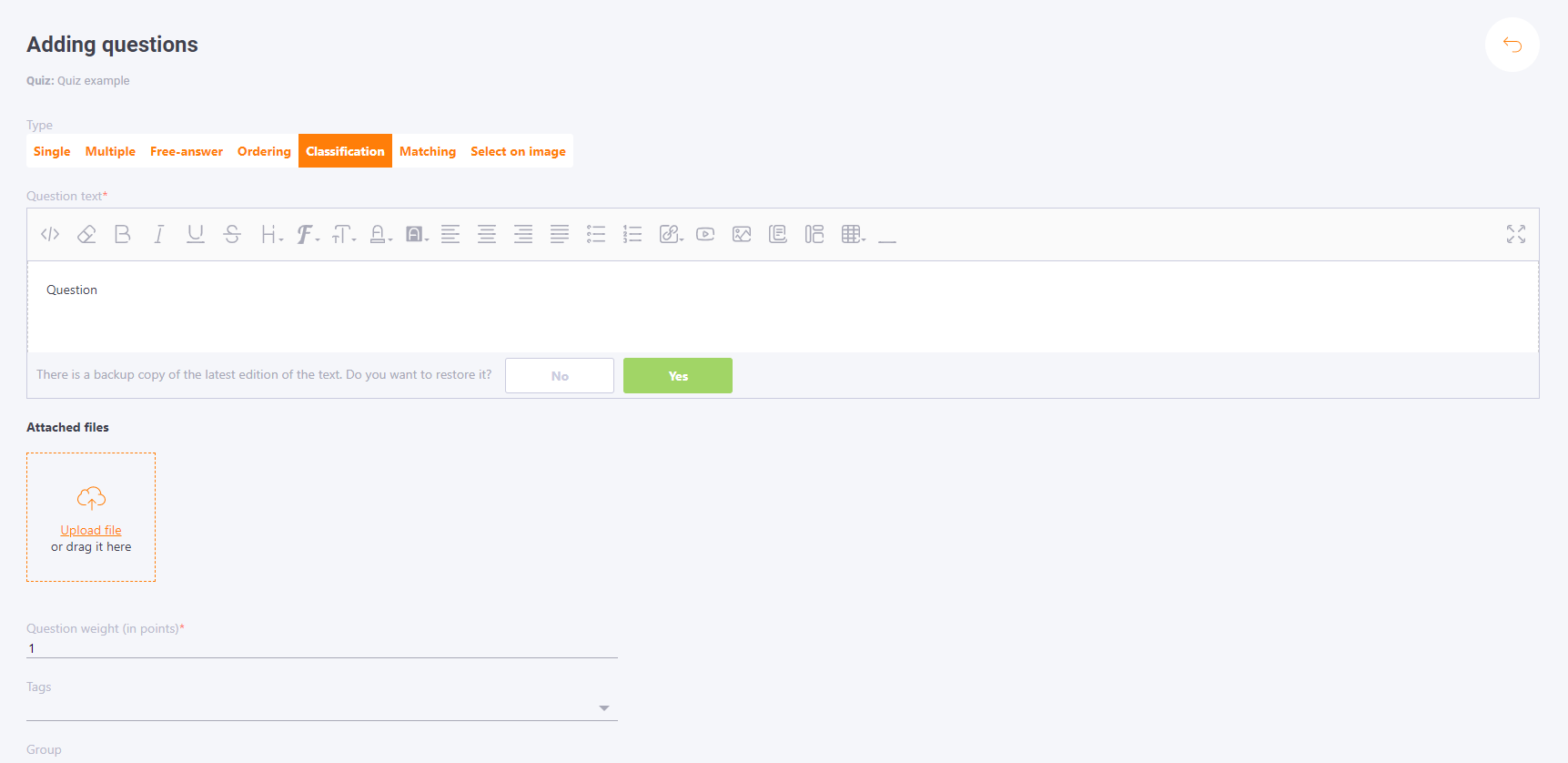First you need to enter the names of categories, then the answer options and which categories they belong to.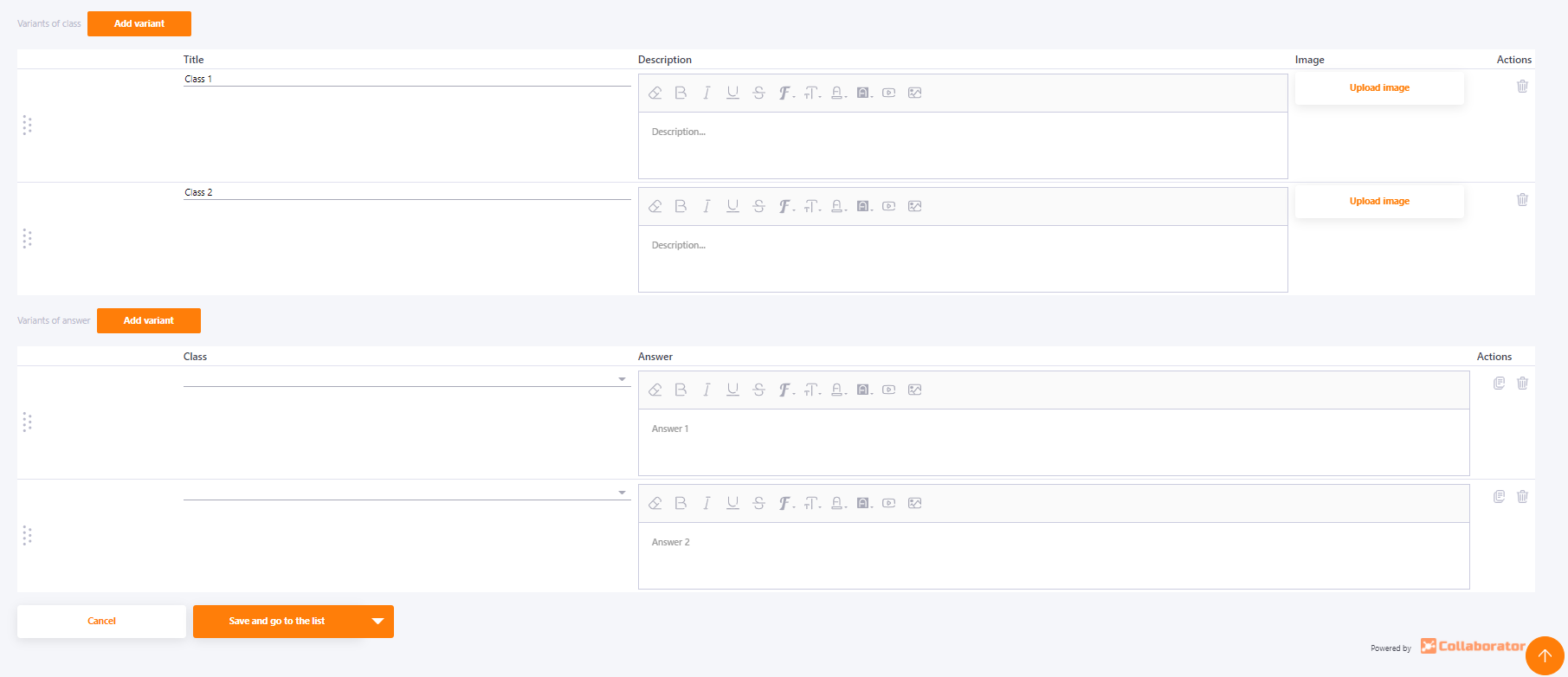#### Matching questions

In the matching questions you need to connect the answer variants with the paired variants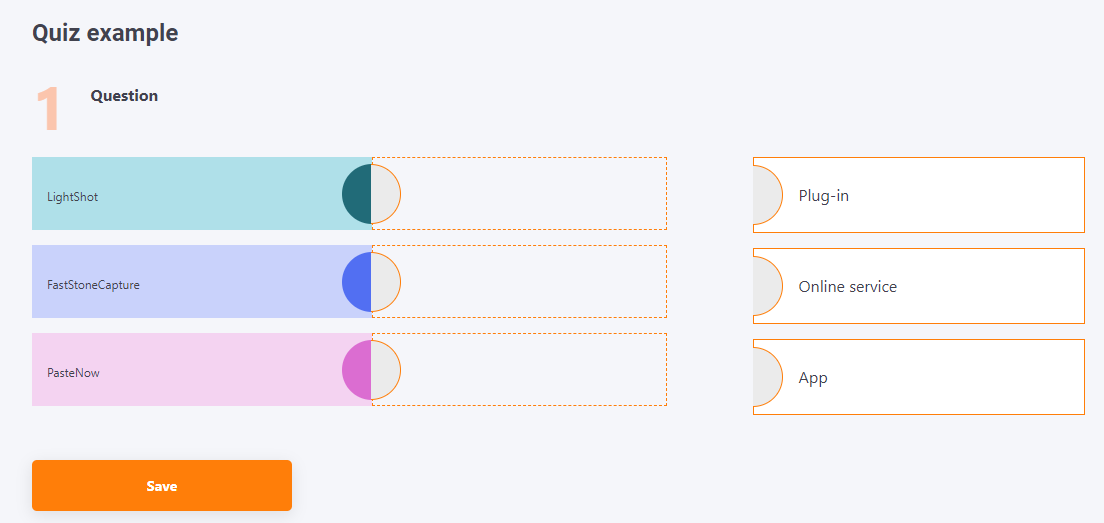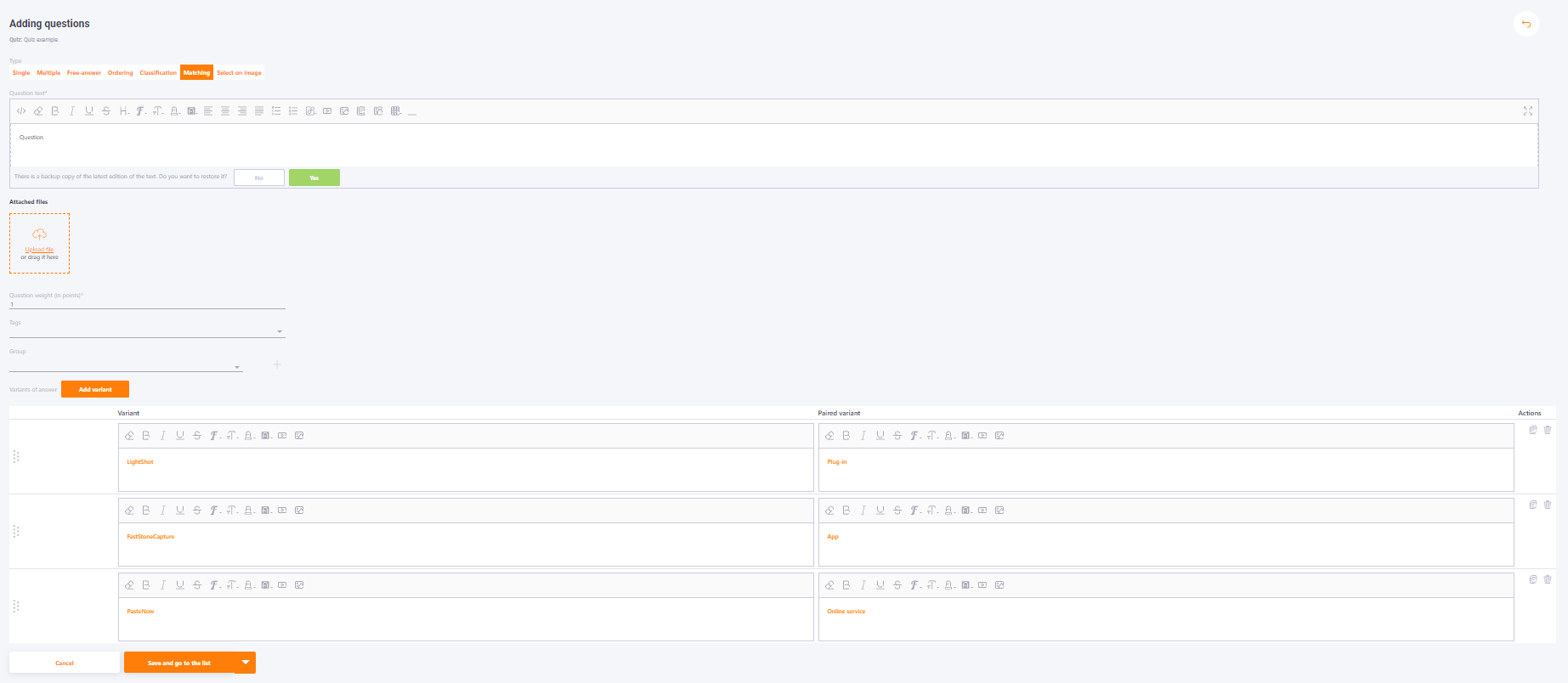#### Select on image questions

The Select on image question type is to select the correct fragment in the image

Question creating

To create a question, you need to fill in the following fields:

• Type - select from the drop-down list the type Select on image
• Question text - editor for creating question text, mandatory field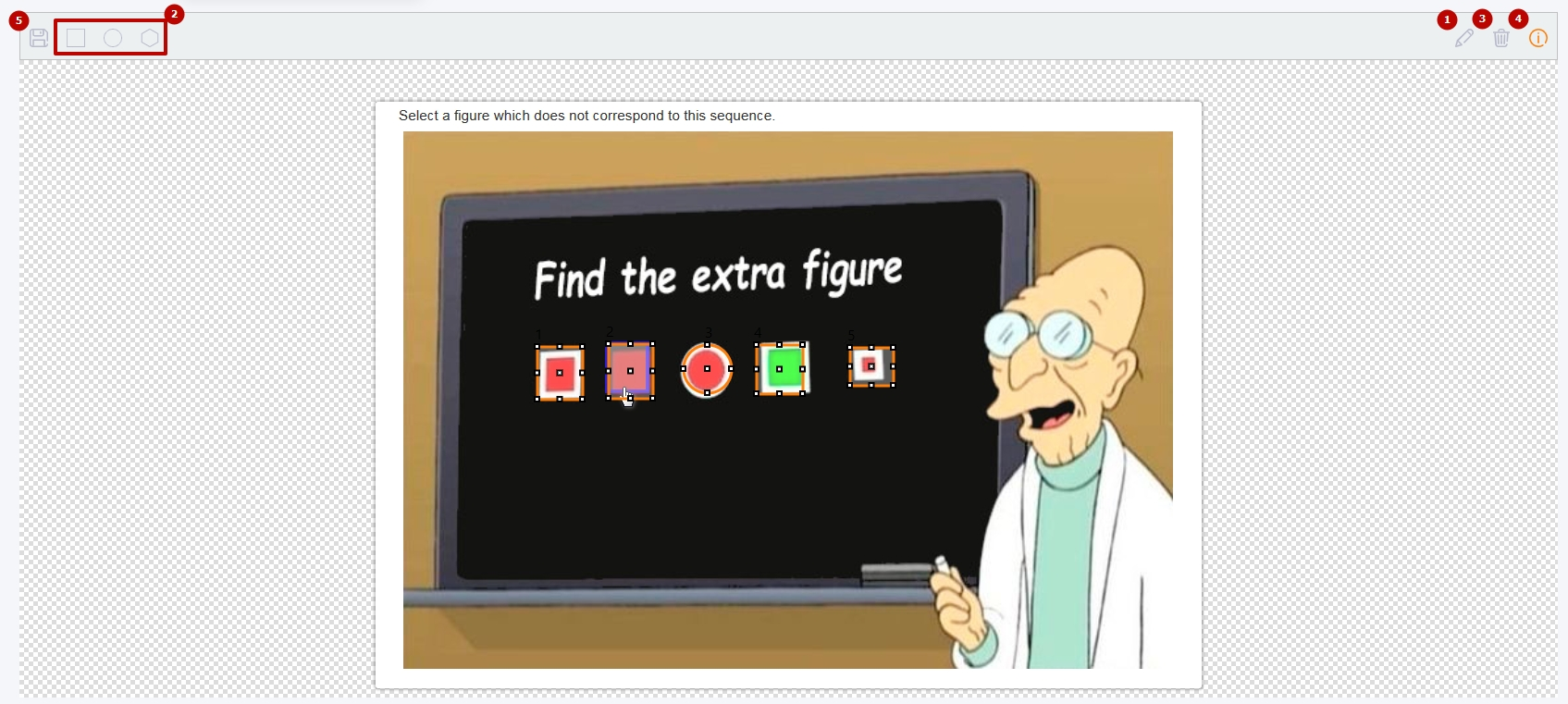1 - the shape of the selection - square, oval or polygonal
2 - edit mode for selected shapes (if the icon is highlighted in the background - enabled) - you can change the size and location of the drawn shapes
3 - clear - deletes all drawn figures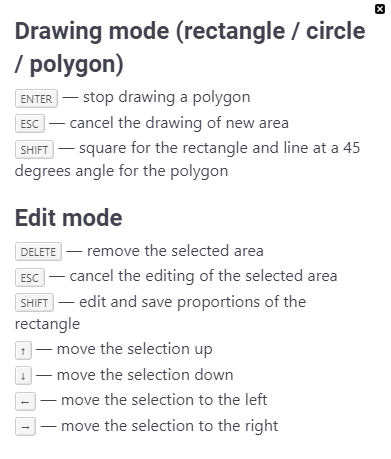After all the necessary fragments on the image have been drawn, you need to save all the answers by clicking on the (5) button.
Each fragment in the picture is marked with a number. These numbers are the same as the answer choices. In the answer choices you have to choose the right one (only one correct choice is available for selection).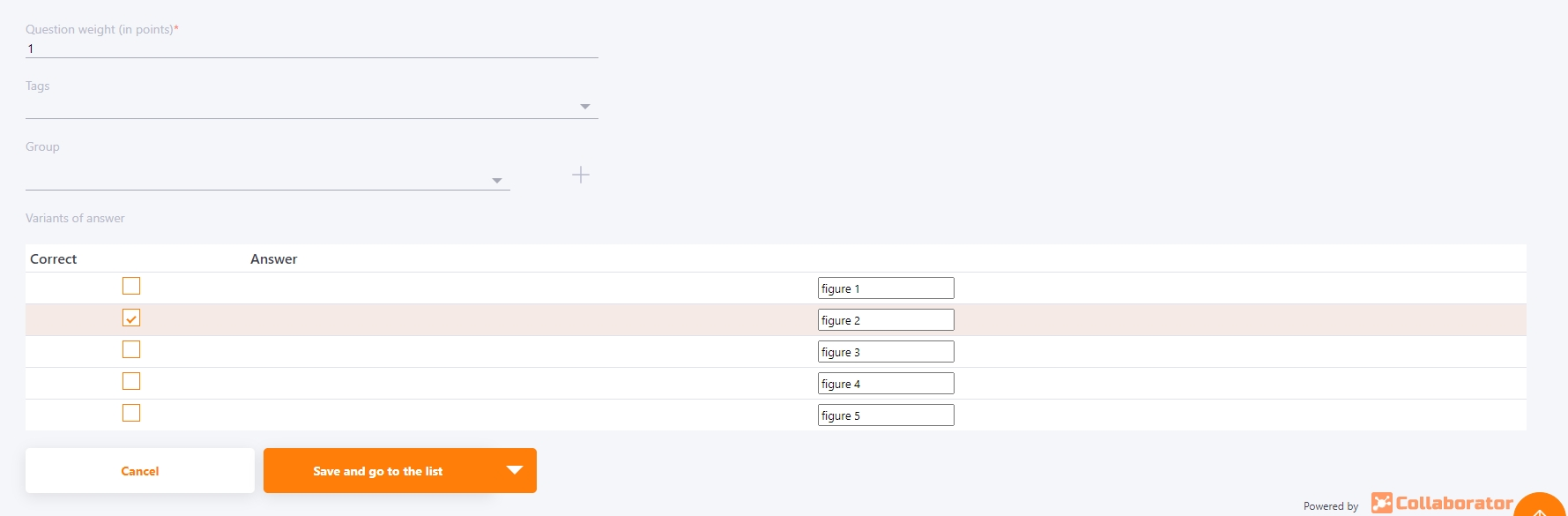If all fields are filled in correctly the button becomes active, a new question can be saved.

Question passing

When you pass a question, only the image is available to the user. When hovering the cursor the fragments are highlighted. The user can select one of these fragments and save the answer.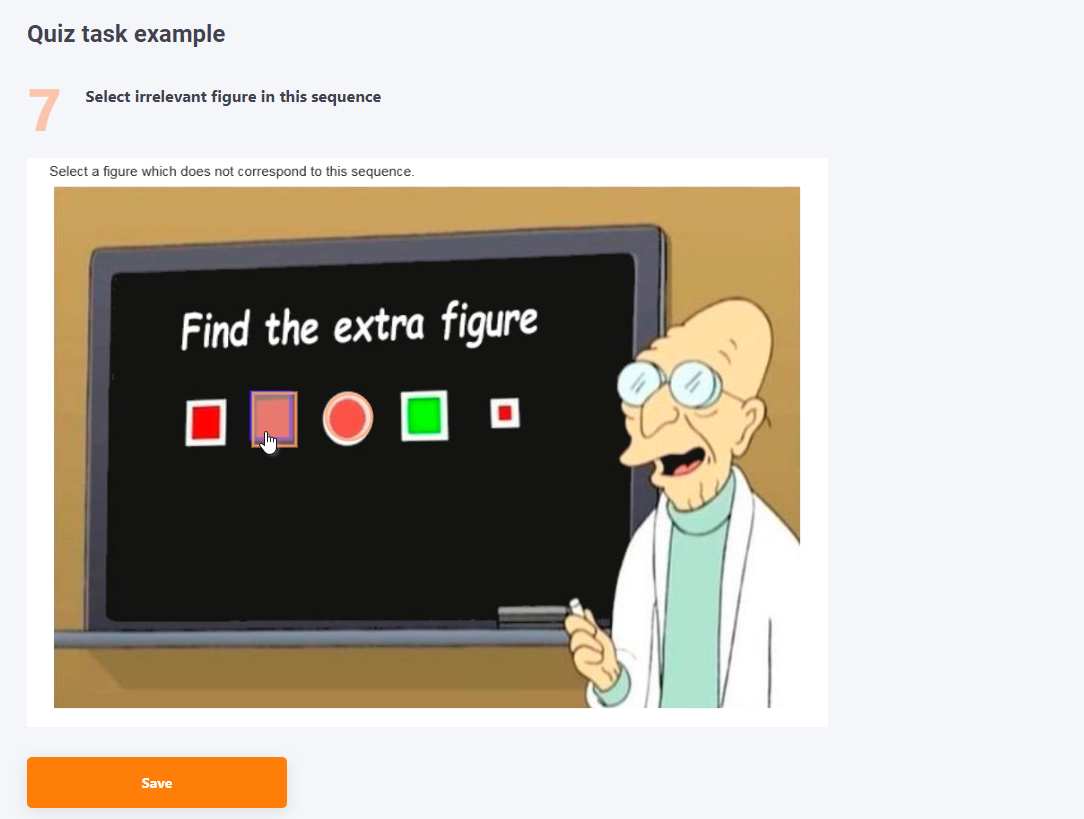Answers checking - mode ( Training mode (allow to check answers for correctness) or Show the user if they gave the right answer to the question)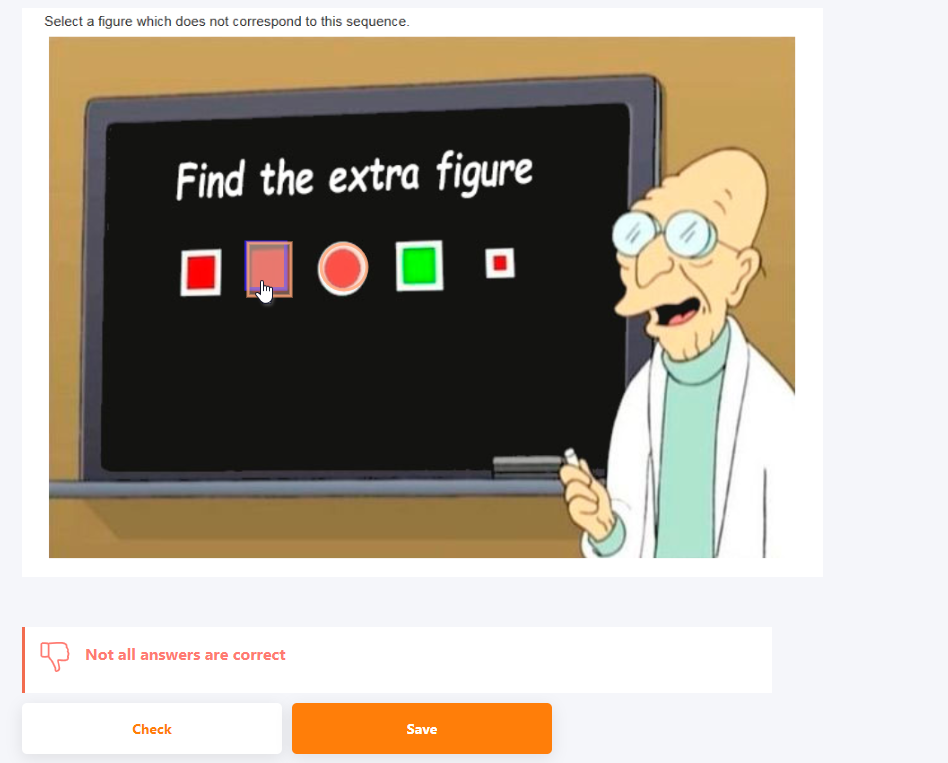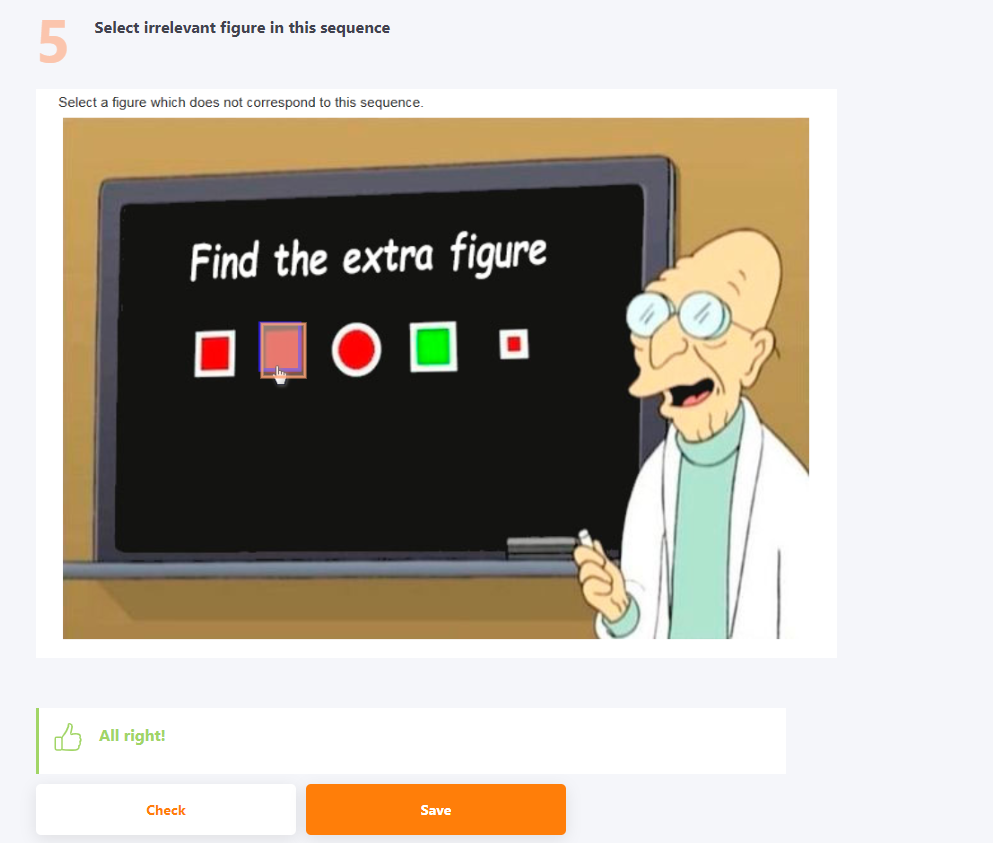Still have questions?
Our technical support team is always happy to help!
Write to us at technical support [email protected]. If you are registered on our Helpdesk - send request button below.
Send request